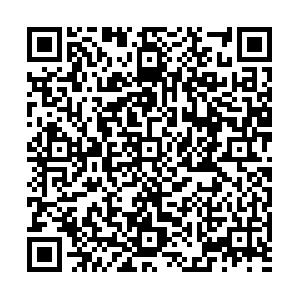# Calculation of Feynman loop integration and phase-space integration via auxiliary mass flow• We extend the auxiliary-mass-flow (AMF) method originally developed for Feynman loop integration to calculate integrals which also involve phase-space integration. The flow of the auxiliary mass from the boundary ($\infty$) to the physical point ($0^+$) is obtained by numerically solving differential equations with respective to the auxiliary mass. For problems with two or more kinematical invariants, the AMF method can be combined with the traditional differential-equation method, providing systematic boundary conditions and a highly nontrivial self-consistency check. The method is described in detail using a pedagogical example of $e^+e^-\rightarrow \gamma^* \rightarrow t\bar{t}+X$at NNLO. We show that the AMF method can systematically and efficiently calculate integrals to high precision.
••Get Citation
Xiao Liu, Yan-Qing Ma, Wei Tao and Peng Zhang. Calculation of Feynman loop integration and phase-space integration via auxiliary mass flow[J]. Chinese Physics C. doi: 10.1088/1674-1137/abc538
Xiao Liu, Yan-Qing Ma, Wei Tao and Peng Zhang. Calculation of Feynman loop integration and phase-space integration via auxiliary mass flow[J]. Chinese Physics C.Milestone
Article Metric

Article Views(162)
Cited by(0)
Policy on re-use
To reuse of Open Access content published by CPC, for content published under the terms of the Creative Commons Attribution 3.0 license (“CC CY”), the users don’t need to request permission to copy, distribute and display the final published version of the article and to create derivative works, subject to appropriate attribution.
###### 通讯作者: 陈斌, bchen63@163.com
• 1.

沈阳化工大学材料科学与工程学院 沈阳 110142

Title:
Email:

## Calculation of Feynman loop integration and phase-space integration via auxiliary mass flow

###### Corresponding author: Peng Zhang, p.zhang@pku.edu.cn
• 1. School of Physics and State Key Laboratory of Nuclear Physics and Technology, Peking University, Beijing 100871, China
• 2. Center for High Energy Physics, Peking University, Beijing 100871, China
• 3. Collaborative Innovation Center of Quantum Matter, Beijing 100871, China

Abstract: We extend the auxiliary-mass-flow (AMF) method originally developed for Feynman loop integration to calculate integrals which also involve phase-space integration. The flow of the auxiliary mass from the boundary ($\infty$) to the physical point ($0^+$) is obtained by numerically solving differential equations with respective to the auxiliary mass. For problems with two or more kinematical invariants, the AMF method can be combined with the traditional differential-equation method, providing systematic boundary conditions and a highly nontrivial self-consistency check. The method is described in detail using a pedagogical example of $e^+e^-\rightarrow \gamma^* \rightarrow t\bar{t}+X$at NNLO. We show that the AMF method can systematically and efficiently calculate integrals to high precision.

### HTMLI.   INTRODUCTIONII.   AUXILIARY MASS EXPANSION AND FLOWIII.   EXAMPLES: MASTER INTEGRALS FOR $e^+e^-\rightarrow \gamma^* \rightarrow t\bar{t}+X$ AT NNLOAPPENDIX A: CALCULATION OF $F^{\rm{cut}}$
Reference (73)
PDF查看关注分享

Top

### 目录/DownLoad:  Full-Size Img  PowerPoint